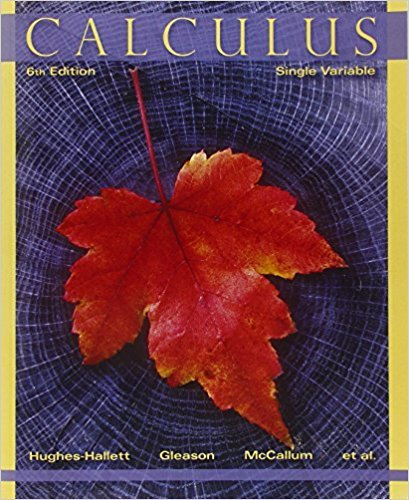×
×

# Solutions for Chapter 9.5: POWER SERIES AND INTERVAL OF CONVERGENCE## Full solutions for Calculus: Single Variable | 6th Edition

ISBN: 9780470888643Solutions for Chapter 9.5: POWER SERIES AND INTERVAL OF CONVERGENCE

Solutions for Chapter 9.5
4 5 0 394 Reviews
24
3
##### ISBN: 9780470888643

This textbook survival guide was created for the textbook: Calculus: Single Variable , edition: 6. Chapter 9.5: POWER SERIES AND INTERVAL OF CONVERGENCE includes 68 full step-by-step solutions. Calculus: Single Variable was written by and is associated to the ISBN: 9780470888643. Since 68 problems in chapter 9.5: POWER SERIES AND INTERVAL OF CONVERGENCE have been answered, more than 32431 students have viewed full step-by-step solutions from this chapter. This expansive textbook survival guide covers the following chapters and their solutions.

Key Calculus Terms and definitions covered in this textbook
• Common difference

See Arithmetic sequence.

• Descriptive statistics

The gathering and processing of numerical information

• Directed angle

See Polar coordinates.

• Ellipsoid of revolution

A surface generated by rotating an ellipse about its major axis

• Explicitly defined sequence

A sequence in which the kth term is given as a function of k.

• Horizontal Line Test

A test for determining whether the inverse of a relation is a function.

• Imaginary part of a complex number

See Complex number.

• Inequality

A statement that compares two quantities using an inequality symbol

• Length of an arrow

See Magnitude of an arrow.

• Local extremum

A local maximum or a local minimum

• nth root

See Principal nth root

• Parallelogram representation of vector addition

Geometric representation of vector addition using the parallelogram determined by the position vectors.

• Pythagorean

Theorem In a right triangle with sides a and b and hypotenuse c, c2 = a2 + b2

• Random behavior

Behavior that is determined only by the laws of probability.

• Reciprocal function

The function ƒ(x) = 1x

• Relation

A set of ordered pairs of real numbers.

• Right angle

A 90° angle.

• Root of a number

See Principal nth root.

• Root of an equation

A solution.

• Triangular number

A number that is a sum of the arithmetic series 1 + 2 + 3 + ... + n for some natural number n.

×